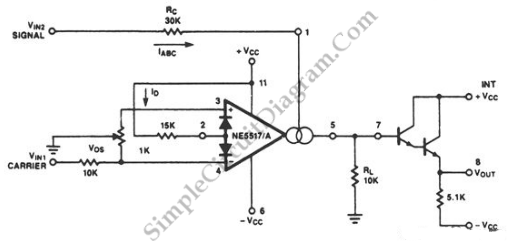# Amplitude Modulator with OTA

Circuit described in the schematic diagram below can achieve 99% modulation easily with maximum frequency of 200kHz. The amplification of this circuit can be controlled easily since the transconductance of an operational transconductance amplifier is directly propotional to the control current Iabc. The product from input voltage times the transconductance is the output current of this circuit. Here is the schematic diagram of the circuit: